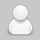## 输出格式

P行，每行一个’Yes’或’No’。表示第i个询问的答案为“具有”或“不具有”亲戚关系。

```6 5 3
1 2
1 5
3 4
5 2
1 3
1 4
2 3
5 6```

```Yes
Yes
No```

## 说明/提示

```// P1551 亲戚 并查集.cpp : 此文件包含 "main" 函数。程序执行将在此处开始并结束。
//

#include <iostream>

using namespace std;
int n, m, p;

int fa;

int find(int);
void hebing(int, int);
int main()
{
cin >> n >> m >> p;
for (int i = 1; i <= n; i++)
{
fa[i] = i;
}
for (int count = 1; count <= m; count++)
{
int a, b;
cin >> a >> b;
hebing(a,b);
}
for (int count = 1; count <= p; count++)
{
int he1, he2;
cin >> he1 >> he2;
int root1, root2;
root1 = find(he1);
root2 = find(he2);
if (root1 == root2)
{
cout << "Yes"<<endl;
}
else
{
cout << "No"<<endl;
}
}
return 0;
}

int find(int x)
{
if (fa[x] == x)
{
return x;
}
else
{
find(fa[x]);
}
}

void hebing(int x, int y)
{
fa[find(x)] = find(y);
}

// 运行程序: Ctrl + F5 或调试 >“开始执行(不调试)”菜单
// 调试程序: F5 或调试 >“开始调试”菜单

// 入门使用技巧:
//   1. 使用解决方案资源管理器窗口添加/管理文件
//   2. 使用团队资源管理器窗口连接到源代码管理
//   3. 使用输出窗口查看生成输出和其他消息
//   4. 使用错误列表窗口查看错误
//   5. 转到“项目”>“添加新项”以创建新的代码文件，或转到“项目”>“添加现有项”以将现有代码文件添加到项目
//   6. 将来，若要再次打开此项目，请转到“文件”>“打开”>“项目”并选择 .sln 文件```

find函数可以这么写：

```int find(int x)
{
if (fa[x] == x)
{
return x;
}
else
{
fa[x] = find(fa[x]);
find(fa[x]);
}
}```

```// P1551 亲戚 并查集.cpp : 此文件包含 "main" 函数。程序执行将在此处开始并结束。
//

#include <iostream>

using namespace std;
int n, m, p;

int fa;

int find(int);
void hebing(int, int);
int main()
{
cin >> n >> m >> p;
for (int i = 1; i <= n; i++)
{
fa[i] = i;
}
for (int count = 1; count <= m; count++)
{
int a, b;
cin >> a >> b;
hebing(a, b);
}
for (int count = 1; count <= p; count++)
{
int he1, he2;
cin >> he1 >> he2;
int root1, root2;
root1 = find(he1);
root2 = find(he2);
if (root1 == root2)
{
cout << "Yes" << endl;
}
else
{
cout << "No" << endl;
}
}
return 0;
}

int find(int x)
{
if (fa[x] == x)
{
return x;
}
else
{
fa[x] = find(fa[x]);
find(fa[x]);
}
}

void hebing(int x, int y)
{
fa[find(x)] = find(y);
}

// 运行程序: Ctrl + F5 或调试 >“开始执行(不调试)”菜单
// 调试程序: F5 或调试 >“开始调试”菜单

// 入门使用技巧:
//   1. 使用解决方案资源管理器窗口添加/管理文件
//   2. 使用团队资源管理器窗口连接到源代码管理
//   3. 使用输出窗口查看生成输出和其他消息
//   4. 使用错误列表窗口查看错误
//   5. 转到“项目”>“添加新项”以创建新的代码文件，或转到“项目”>“添加现有项”以将现有代码文件添加到项目
//   6. 将来，若要再次打开此项目，请转到“文件”>“打开”>“项目”并选择 .sln 文件```

标签： 并查集

非特殊说明，本文版权归 Chuanrui の 初见之旅 所有，转载请注明出处.

本文分类： NOIP 2021

本文标题： P1551 亲戚

###### 延伸阅读
• 评论列表
•发布于2021-06-07 10:37:17
• 感谢分享 赞一个

发表评论:

◎欢迎参与讨论，请在这里发表您的看法、交流您的观点。